# Electric Forces Coulombs Law Group Problems What is

• Slides: 11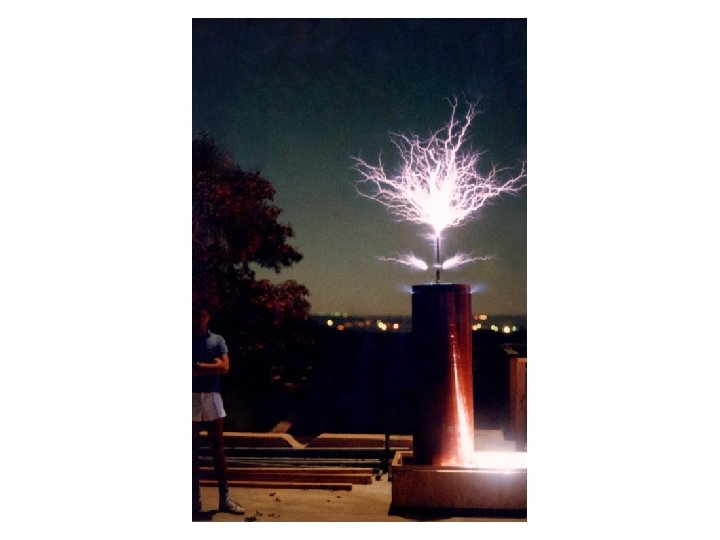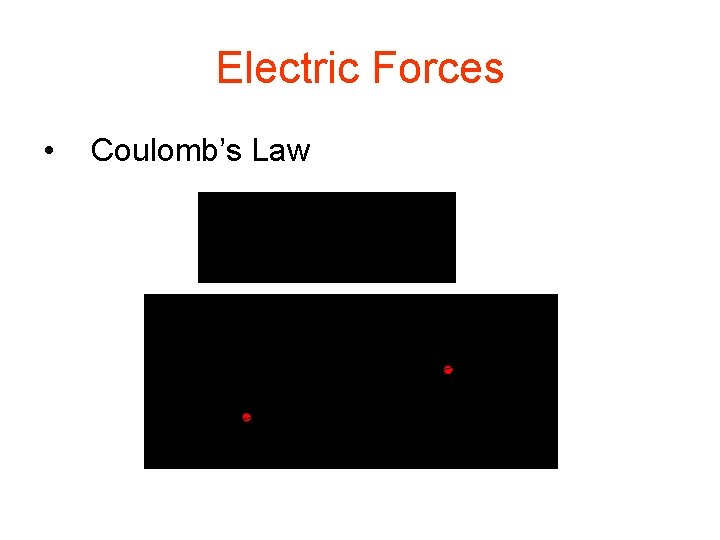Electric Forces • Coulomb’s LawGroup Problems What is the force (magnitude and direction) on a -10 m. C charge located at x = 4 m from a 5 m. C charge at the origin? Steps: a. Draw quick sketch (labeled with info) b. Decide on relevant idea/theory/equation and make correspondence of variables – what is given, what is unknown – can you solve for unknowns? c. Do the math – be sure to answer completely and to put units d. Check answer if at all possible 1. 2. Repeat the previous problem when the 5 m. C charge is located at x = 1 m and is replaced with a -5 m. C charge. 3. What is the force on a charge Q located at x 1 from a charge q located at x 2? Try to get a general expression that works for all values of the variables.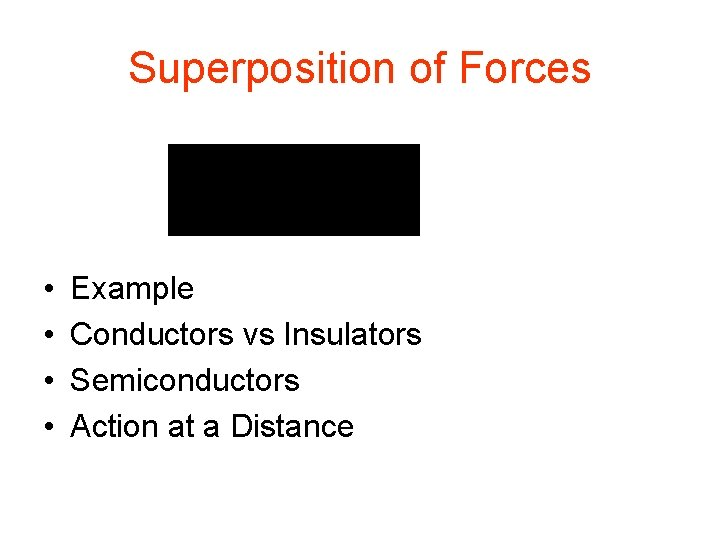Superposition of Forces • • Example Conductors vs Insulators Semiconductors Action at a Distance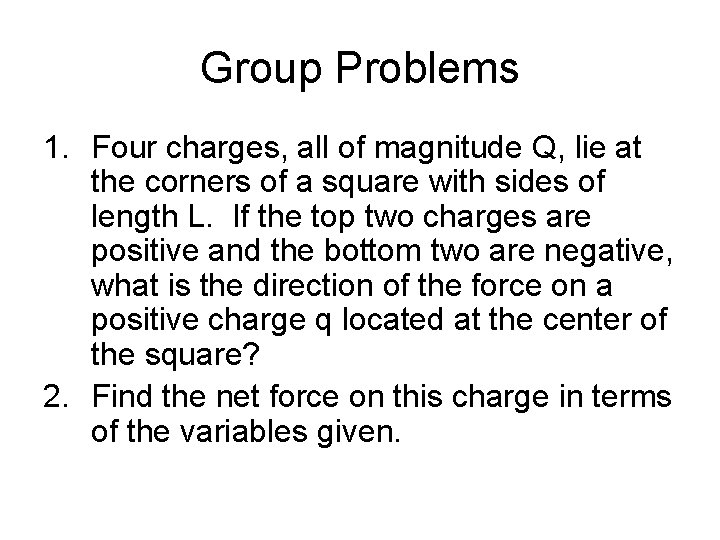Group Problems 1. Four charges, all of magnitude Q, lie at the corners of a square with sides of length L. If the top two charges are positive and the bottom two are negative, what is the direction of the force on a positive charge q located at the center of the square? 2. Find the net force on this charge in terms of the variables given.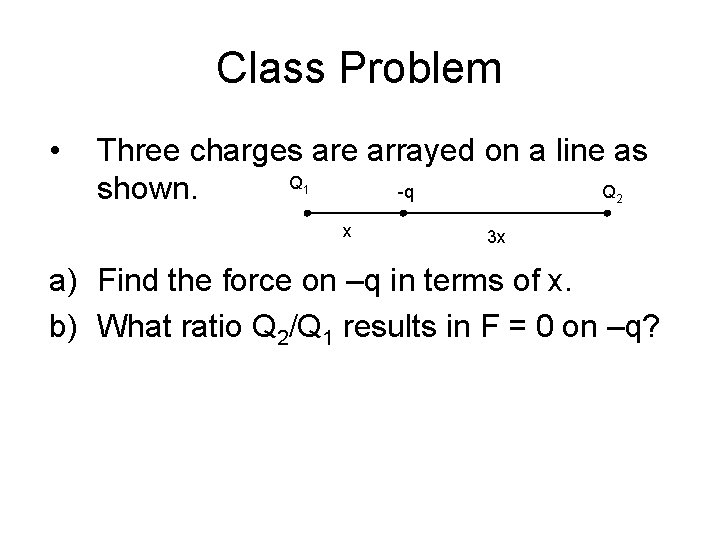Class Problem • Three charges are arrayed on a line as Q -q Q shown. 1 2 x 3 x a) Find the force on –q in terms of x. b) What ratio Q 2/Q 1 results in F = 0 on –q?Electric Field E field of point charge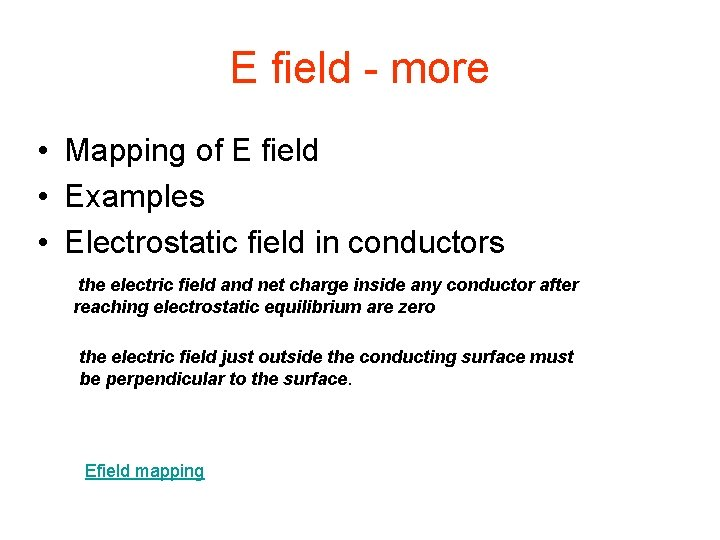E field - more • Mapping of E field • Examples • Electrostatic field in conductors the electric field and net charge inside any conductor after reaching electrostatic equilibrium are zero the electric field just outside the conducting surface must be perpendicular to the surface. Efield mapping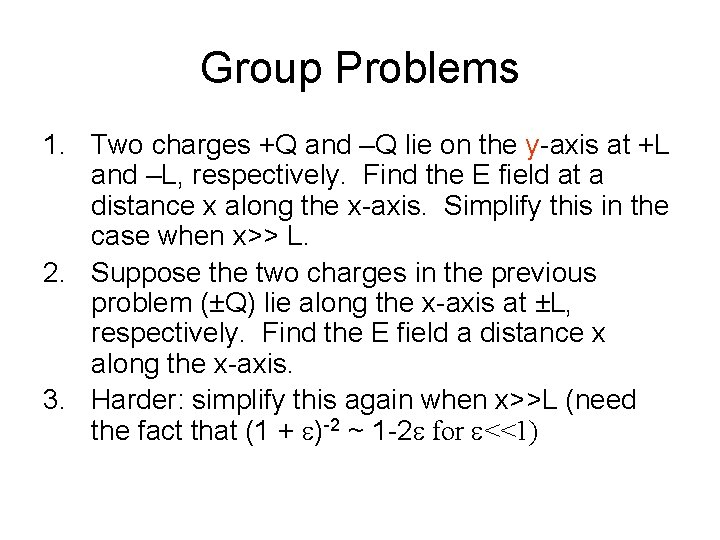Group Problems 1. Two charges +Q and –Q lie on the y-axis at +L and –L, respectively. Find the E field at a distance x along the x-axis. Simplify this in the case when x>> L. 2. Suppose the two charges in the previous problem (±Q) lie along the x-axis at ±L, respectively. Find the E field a distance x along the x-axis. 3. Harder: simplify this again when x>>L (need the fact that (1 + e)-2 ~ 1 -2 e for e<<1)Electrophoresis • • Physics Macromolecular charges Problems Modern Methods - PAGE - Isoelectric focusing